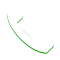## Time Series Best Practices

Producing statistically robust results with univariate time-series data requires following a few best practices and methodologies. Many programming languages such as R, MATLAB and Python include packages which allow the end user to employ a variety of statistical tests, nullifying the need to write complex functions. The following models represent a handful of common methodologies used for forecasting.

Autoregressive (AR) and Moving Average (MA) Models:

For brevity, formulae explaining each model will not be included in this post. The first step in working with time-series data involves checking if the series is stationary (i.e., displaying a constant mean and variance over time). A quick visual check can often give an idea of a stationary series (e.g., does the series appear to be mean reverting as in Figure 1?), however, best practices would entail running an Augmented Dickey Fuller (ADF) test for a unit root on the series1. A unit root being present in a series can cause problems in performing regression analyses, such as spurious regressions2 which indicate strong relational patterns between variables when they do not actually exist in practice. If the p-value of the ADF test falls below the significance level (α) that was specified, the series is said to be stationary. If not, one must difference the series until the p-value falls below α.

Figure 1) Logged returns depicting stationarity (confirmed by an ADF test) – notice the appearance of a “horizontal” trend

Autoregressive models have lag lengths denoted p and are used for forecasting if the user believes that the series will be dependent on lagged data points. Moving average models have lag lengths denoted by q and are used if there is belief that the sum of the error term/residuals (at) are to influence the series. ARMA models (lags p and q) are also popular in econometrics and volatility modelling, as they represent a combination of both AR and MA models and allow for a more flexible structure.

Figure 2) A plot examining an ARMA(1,1) model’s fit and residual plot with means centered around zero

To determine the optimal lag length in the abovementioned models, Akaike (AIC) and Swartz-Bayesian (BIC) criterions may be used. The difference between the criterions is that the BIC penalizes for larger sample sizes and suggests a lower number of lags compared to the AIC criterion3. To determine the number of lags p and q, find the minima of the AIC and BIC criteria. A useful test to check if the lag length selection should be increased is to run a Ljung-Box test on the residual series, which checks if the residuals follow a white noise process – if the null of this test is rejected, one should increase the lag length selection4. The Ljung-Box test is used to examine the “fit” of the residuals – if the null hypothesis is rejected, the model is said to exhibit “lack” of fit. The end goal is to choose the correct number of lags so that the residual series is a white noise series, which occurs when the error terms/residuals are independently and identically distributed (i.i.d).

ARCH and GARCH Models:

ARCH models differ from ARMA models in that they allow the variance of the previous error terms to be non-constant (i.e., time-varying), whereas with ARMA models the error terms have constant conditional past variances. ARCH models are useful as they can generate fat tails commonly observed in return series, differing from the standard normal distribution. ARCH models should be used if the residual series demonstrates periods of clustered volatility – think low volatility for a while, then high volatility which evolves continuously5.

Testing for an ARCH effect uses the residuals of the series (at) to see if the squared series (at2) displays serial correlation, which is the relation between the variable of interest and the lagged variable. It is ideal to use a Ljung-Box test to check whether the autocorrelation functions of the residual series are different from zero or not, which can tell the user if conditional heteroskedasticity is present4. A real-world example of GARCH models is their use in estimating Value at Risk (VaR), where one calculates the probability of a loss being above a certain threshold for a period. Whilst this was only a miniscule portion of some best practices to work with time-series data, following the abovementioned steps will result in a higher degree of confidence for forecasting work.

References:

1. Unit root: Simple definition, unit root tests. Statistics How To. (2021, January 1). Retrieved November 29, 2022, from //www.statisticshowto.com/unit-root/
2. Lecture 8A: Spurious regression – Miami University. (n.d.). Retrieved November 29, 2022, from //www.fsb.miamioh.edu/lij14/672_2014_s8.pdf
3. Brownlee, J. (2020, August 27). Probabilistic model selection with AIC, BIC, and MDL. MachineLearningMastery.com. Retrieved November 29, 2022, from //machinelearningmastery.com/probabilistic-model-selection-measures/
4. 2 Diagnostics: Stat 510. PennState: Statistics Online Courses. (n.d.). Retrieved November 29, 2022, from //online.stat.psu.edu/stat510/lesson/3/3.2
5. ARCH Models. Jing Li. (n.d.). Retrieved November 29, 2022, from //www.fsb.miamioh.edu/lij14/

## Connect with Sapling Financial ConsultantsCall Now